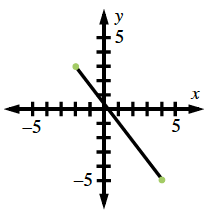### Home > PC > Chapter 3 > Lesson 3.1.1 > Problem3-11

3-11.

Write an equation for the perpendicular bisector of the segment joining $A\left(−2, 3\right)$ and $B\left(4, −5\right)$ in point-slope form.

Graph the points and draw the segment between them.Calculate the slope of the line between the points.
$m = \frac{y_2 - y_1}{x_2 - x_1}$

Then find negative the reciprocal (flip the fraction) of this slope for the perpendicular slope.

Find the midpoint.
$(\frac{x_2 + x_1}{2} + \frac{y_2 + y_1}{2})$

Enter the information found:
Point-slope form: $y − y_{1} = m\left(x − x_{1}\right)$